# Metal Weight Calculator

Use this metal weight calculator to calculate the weight of beams, bars, sheets, columns, tubings, etc. made from steel, aluminum, nickel, iron, copper and other commonly used metals. Versatile online steel weight calculator.

g/cm3

### Calculation results

1.499 kg (1,498.540 g) Unit mass Total mass
Share calculator:

Embed this tool:
get code

## Metal weight calculation

Calculating the weight of any type of metal product: beams, profiles of various types, bars, tubes, pipes, sheets, etc. is similar to that of any other type of material. We need to know the volume of the metal (cu in, cu mm, cu cm, etc.) and its density (usually in g/cm3, oz/in3). Multiplying the two gives us the resulting metal weight.

In the metal weight calculator above we have pre-entered the densities of many commonly used metals like carbon steel, stainless steel, iron, copper, nickel, aluminum, as well as metal alloys such as bronze, aluminum and nickel alloys: Nickel 200, Monel 400, Inconel 600, Inconel 625, Inconel 718, Inconel X-750, Incoloy 800. Selecting a material from the list will automatically fill in its density in the "density" field. However, you can always enter a custom density, if it is known to you and the calculator will use that instead. A common mistake is to confuse "steel" with "metal". The two are not synonyms! In fact, steel is not technically a metal, but a metal alloy, since it is made from a mixture of iron, carbon, and other elements in very small quantities.

The calculator has a set of different types of products to choose from, and each would require a different set of measurements to be entered, in order to calculate its volume.

## Types of metal products supported in the metal calculator

Our steel weight calculator supports different types of products, as described below. If you wish to calculate a more complex product, you can either break it down into simpler components which you can then calculate individually, or use a more advanced piece of software.

### Round metal bar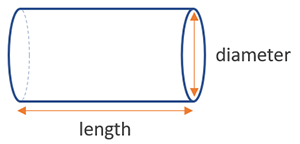A round metal bar is one of the easiest ones to calculate, as the weight equation requires only 2 measurements to be known: the diameter and the length of the bar. For example, let's calculate the weight in steel of a bar with length 1 meter and diameter of 20 mm.

The volume of the steel bar is the product of the area of the cross-section and the length: π x r2 x l = 3.1416 x 102 x 1000 = 314,160 mm3 = 314.16 cm 3 (r = 1/2 x diameter, l = 1 m = 1000 mm). If using a carbon steel with density of 7.95 g/cm3, we need the product of 7.95 and 314.16, which equals 2497.572 g, or ~2.498 kg.

### Rectangular or square metal bar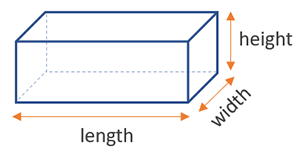In order to calculate the weight of a square metal bar you only need to know one side of its cross-section and its length, but you will still need to enter two sides, since our tool also supports rectangular bars for which the second side can differ. The formula then is to multiply the three together: width x height x length, and then multiply by the density to get to the weight. For example, for an aluminum rectangular bar with a cross section of 20 mm by 30 mm and a length of 2 meters, we need to calculate the volume as 20 x 30 x 2000 = 1,200,000 cubic millimeters or 1,200 cubic centimeters. Given a density of 2.72, we then get the product of 2.72 x 1,200 = 3,264 g or 3.264 kg.

### Hexagonal metal bar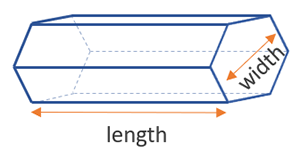Two measurements are needed as input to the metal weight calculator for a hexagonal bar: length and width, where the width is the distance between any two of its opposing sides. We only support regular hexagons for the moment. Given the width we can easily calculate the area of the hexagon cross-section and from there - the total volume and weight of the bar.

### Metal sheet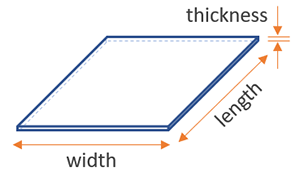A metal sheet is no different than a rectangular bar, it is in the steel weight calculator for your convenience mostly. The formula used to compute the weight is exactly the same.

### Metal tubing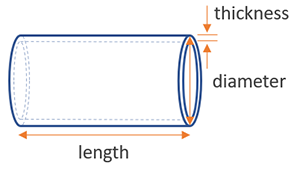The weight of a metal tube or pipe is a bit more complex to compute than that of a round metal bar, since we need to know either both the inner and outer diameters, or one of the diameters and the thickness of the tubing. We chose to require the outer diameter and the thickness, as these are usually the easiest to measure (and if you have the plans in front of you, it should be easy to get any two numbers either way).

### Rectangular metal profile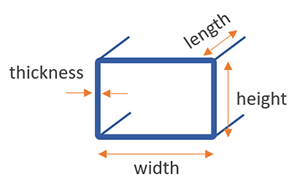Rectangular metal profiles are used very often in construction due their good ability to resist forces from all directions. We currently only support purely rectangular profiles with straight angles only. The added dimension in comparison to a rectangular bar is the profile thickness. The metal weight is again simply the volume times the metal density.

### L-profile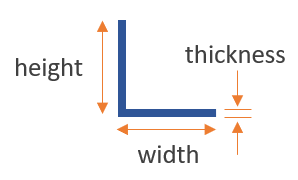The l (small L) profile is just two metals planks wielded or cast together, at an angle of 90 degrees. It is basically a base and a flange on just one side. We support metal weight calculations for metal angles with equal or unequal arms.

### U-profile (U-channel, C-channel)

Weight calculations for these metal profiles require a lot of measurements to be known due their complex shape. The U-profile, also known as U-channel in Europe and as C-channel in the US, is shown below: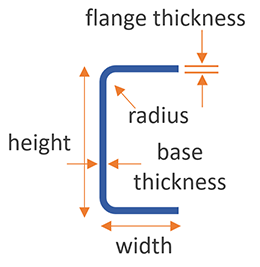The U profile has a base and two parallel flanges: one on either side of it. They are also called U-channels and a lot of them are standardized. Our steel calculator has many of the standard profiles - the EU UPN and UPE, the U.S. C and BC channels, so you can just select them and we will use their details automatically. Otherwise you can specify a custom profile.

Below you see an illustration of the UPN and UPE channels, which are equivalent in overall shape to C-channels and BC-channels, respectively.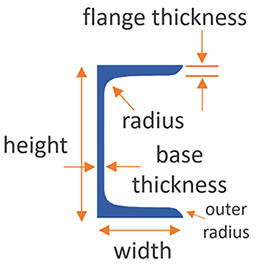UPN steel profiles are used widely in many industrial applications and machinery building. C-Channels are their american standardized equivalent.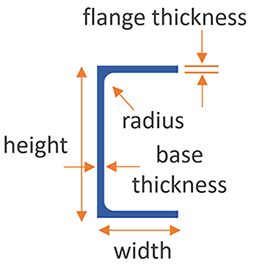UPE profiles have thinner thickness, but slightly wider flanges than UPN profiles and comparable static values. Using UPE profiles can potentially result in weight saving of up to 30% with barely any sacrifice in static conditions.

BC is short for "Bar Channel section with parallel flanges". Its flanges and webs have the same thickness by definition and they are often used as a low-cost alternative to heavier profiles for a broad variety of applications as the specific weight per meter value is relatively low.

IPE and BC channels are significantly easier to assemble as there is no need for conical flat washers to compensate the natural conicity of the C-Channel / UPN channel flanges.

### I-profile (H-profile, I-beam, or H-beam)

The I-profile, also known as an I-beam, H-profile, and H-beam, are like two U-channels, back to back. It has two flanges and a web between them. There are two types of shapes for the cross-section of an I-beam. The W-shaped has flanges that have about the same thickness from end to end, while the S-shaped has flanges that are significantly thinner near their edges. Both are used as support beams for construction, facilities and engineering, factory shops, warehouses and truck bed framing. Our online tool supports weight calculation of I-profiles with any dimensions.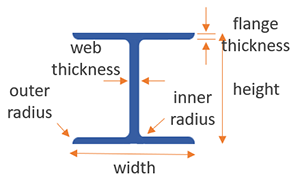There are way too many standards of such profiles, such as IPE, IPN, HD, HE, HL, HP, S, and so on. Due to the sheer number of standards we don't have automatic fill-in for these types, you would need to type the numbers into the calculator yourself and calculations for S-shaped beams are likely to be less accurate than those of W-shaped ones.

### Metal T-profile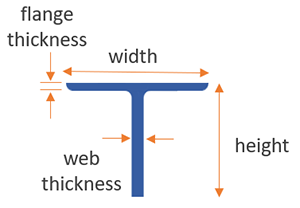A T-profile is just as it sounds: it looks like the letter T. It can be a jointed one - if it is welded or bolted, or warm-manufactured - when it's hot rolled or extruded. As with other profiles and beams the horizontal part of the cross-section is called a "flange" while the vertical part is called "web". Our calculator supports metal weight calculations for all kinds of custom T-beams.

Steel bridge